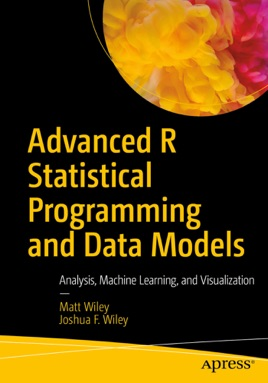• \$39.99

## Publisher Description

Carry out a variety of advanced statistical analyses including generalized additive models, mixed effects models, multiple imputation, machine learning, and missing data techniques using R. Each chapter starts with conceptual background information about the techniques, includes multiple examples using R to achieve results, and concludes with a case study.
Written by Matt and Joshua F. Wiley, Advanced R Statistical Programming and Data Models shows you how to conduct data analysis using the popular R language. You’ll delve into the preconditions or hypothesis for various statistical tests and techniques and work through concrete examples using R for a variety of these next-level analytics.  This is a must-have guide and reference on using and programming with the R language.
You will:
Conduct advanced analyses in R including: generalized linear models, generalized additive models, mixed effects models, machine learning, and parallel processing
Carry out regression modeling using R data visualization, linear and advanced regression, additive models, survival / time to event analysis
Handle machine learning using R including parallel processing, dimension reduction, and feature selection and classification
Address missing data using multiple imputation in R
Work on factor analysis, generalized linear mixed models, and modeling intraindividual variability